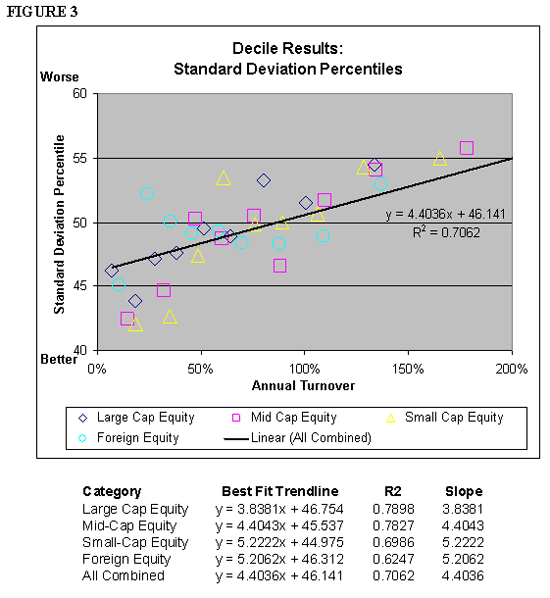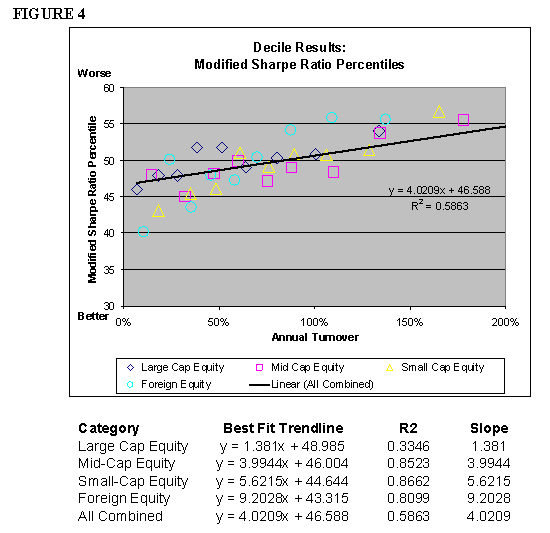# The Pre-Tax Costs Of Portfolio Turnover

May 01, 2007

Standard Deviation Percentile Results

Figure 3 contains the percentile standard deviations for each of the four test groups. Unlike the previous outperformance chart, where the raw outperformance values were used, the standard deviation results were considered on a percentile basis. Using percentiles was important because the standard deviations varied considerably among the six different (three-year) test periods, and because standard deviations cannot be combined as easily as raw outperformance (i.e., taking the average is not a correct way to combine them). Similar to the deciles, lower percentiles are considered to be better than higher percentiles. Below the chart is a table that lists the linear best-fit trend lines, coefficient of determination (R²) and the slopes of the best-fit trend line for each of the groups tested. The exact figures are included in Figure 5.Those investments with lower turnover rates tended to have lower standard deviations over the test period. Therefore, higher turnover can typically be associated with higher levels of variability, or risk. Similar to the outperformance results, small capitalization equities were more (negatively) affected by turnover than large capitalization equities, as can be seem from the slopes.

Sharpe Ratio Percentile Results

Figure 4 contains the Modified Sharpe Ratio results for each of the four test groups for each decile. Similar to the standard deviation results, these results are included on a percentile basis, where 1 is considered the best and 100 the worst. Below the chart is a table that lists the linear best-fit trend lines, coefficient of determination (R²) and the slopes of the best-fit trend line for each of the groups tested. The exact figures are included in Figure 5.As can be expected, since both outperformance and risk (as defined by standard deviation) were better for funds with lower turnover, those funds which tended to have lower turnover had higher Modified Sharpe Ratios than those funds with higher turnover. Again, the slope (i.e., the impact of turnover) increased for smaller capitalization levels. While Bauman, Miller and Veit (2005) contend that higher turnover can lead to higher risk-adjusted performance, the research conducted for this paper suggests this is not the case.